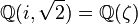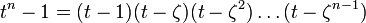# Linear representation theory of finite cyclic groups

This article gives specific information, namely, linear representation theory, about a family of groups, namely: cyclic group.
View linear representation theory of group families | View other specific information about cyclic group

This article discusses the linear representation theory of a finite cyclic group of order$n$, which for concreteness we take as the group of integers modulo n, i.e., the group$\mathbb{Z}/n\mathbb{Z}$ or$\mathbb{Z}_n$.

For the linear representation theory of the infinite cyclic group, see linear representation theory of group of integers.

## Summary

We denote by$\varphi(n)$ the Euler totient function of$n$, defined as the number of numbers in$\{1,2,\dots,n \}$ that are relatively prime to$n$, or equivalently as the order of the multiplicative group of the ring of integers modulo$n$.

Item Value
degrees of irreducible representations over a splitting field 1 ($n$ times)
maximum: 1, lcm: 1, number:$n$, sum of squares:$n$
Schur index values of irreducible representations 1 ($n$ times)
smallest ring of realization of all irreducible representations$\mathbb{Z}[\zeta]$ where$\zeta$ is a primitive$n^{th}$ root of unity. This is a degree$\varphi(n)$ integral extension of$\mathbb{Z}$.
minimal splitting field (smallest field of realization of all irreducible representations) in characteristic zero$\mathbb{Q}(\zeta)$ where$\zeta$ is a primitive$n^{th}$ root of unity. This is a degree$\varphi(n)$ cyclotomic Galois extension of$\mathbb{Q}$.
condition for field to be a splitting field characteristic does not divide$n$, must contain a primitive$n^{th}$ root of unity
minimal splitting field in characteristic$p$ that does not divide$n$ Field of size$p^r$ where$n$ divides$p^r - 1$.
smallest size splitting field smallest prime power$q$ such that$n$ divides$q - 1$.
degrees of irreducible representations over the reals Case$n$ odd: 1 (1 time), 2 ($(n - 1)/2$ times)
Case$n$ even: 1 (2 times), 2 ($(n - 2)/2$ times)
degrees of irreducible representations over the rationals For every positive divisor$d$ of$n$, there is one representation of degree$\varphi(d)$ (where$\varphi$ is the Euler totient function)
maximum:$\varphi(n)$, lcm:$\varphi(n)$, number:$\tau(n)$ where$\tau$ is the divisor count function

## Particular cases$n$ cyclic group linear representation theory page degrees of irreducible representations over splitting field degrees of irreducible representations over reals degrees of irreducible representations over rationals smallest splitting field (characteristic zero) smallest size of finite splitting field
1 trivial group -- 1 1 1$\mathbb{Q}$ 2, i.e., field:F2
2 cyclic group:Z2 linear representation theory of cyclic group:Z2 1,1 1,1 1,1$\mathbb{Q}$ 3, i.e., field:F3
3 cyclic group:Z3 linear representation theory of cyclic group:Z3 1,1,1 1,2 1,2$\mathbb{Q}(\zeta)$ where$\zeta$ is a primitive cube root of unity 4, i.e., field:F4
4 cyclic group:Z4 linear representation theory of cyclic group:Z4 1,1,1,1 1,1,2 1,1,2$\mathbb{Q}(i)$ where$i$ is a square root of$-1$ 5, i.e., field:F5
5 cyclic group:Z5 linear representation theory of cyclic group:Z5 1,1,1,1,1 1,2,2 1,4$\mathbb{Q}(\zeta)$ where$\zeta$ is a primitive fifth root of unity 11, i.e., field:F11
6 cyclic group:Z6 linear representation theory of cyclic group:Z6 1,1,1,1,1,1 1,1,2,2 1,1,2,2$\mathbb{Q}(\zeta)$ where$\zeta$ is a primitive cube root of unity 7, i.e., field:F7
7 cyclic group:Z7 linear representation theory of cyclic group:Z7 1,1,1,1,1,1,1 1,2,2,2 1,6$\mathbb{Q}(\zeta)$ where$\zeta$ is a primitive seventh root of unity 8, i.e., field:F8
8 cyclic group:Z8 linear representation theory of cyclic group:Z8 1,1,1,1,1,1,1,1 1,1,2,2,2 1,1,2,4$\mathbb{Q}(i,\sqrt{2}) = \mathbb{Q}(\zeta)$ where$\zeta$ is a primtive eighth root of unity 9, i.e., field:F9
9 cyclic group:Z9 linear representation theory of cyclic group:Z9 1 (9 times) 1,2,2,2,2 1,2,6$\mathbb{Q}(\zeta)$ where$\zeta$ is a primitive ninth root of unity 19, i.e., field:F19

## Group ring interpretation

For any commutative unital ring$R$, the group ring$R[\mathbb{Z}_n]$ is isomorphic to the ring:$R[t]/(t^n - 1)$

where the isomorphism sends an element$a \pmod n$ to the equivalence class of$t^a$.

### Case of ring that contains primitive roots of unity and is uniquely$n$-divisible

If$R$ contains a primitive$n^{th}$ root of unity, say$\zeta$, then we have:$t^n - 1 = (t - 1)(t - \zeta)(t - \zeta^2) \dots (t - \zeta^{n-1})$

If$R$ is uniquely$n$-divisible, then by the Chinese remainder theorem, we get the following direct sum/direct product decomposition as rings:$R[t]/(t^n - 1) \cong R[t]/(t - 1) \times R[t]/(t - \zeta) \times R[t]/(t - \zeta^2) \times \dots \times R[t]/(t - \zeta^{n-1}) \cong R \times R \times R \times \dots \times R$

(We could also use$\oplus$ instead of$\times$ in the line above).

The choice of decomposition using the Chinese remainder theorem also corresponds to the use of characters, as follows. We have an isomorphism:$R[t]/(t^n - 1) \cong Re_1 \oplus Re_2 \oplus \dots Re_n$

where the$e_j$ are idempotents. Each idempotent is given as follows: take a character, and consider the element of the group ring obtained by multiplying each group element by its character value, and dividing the sum by the order of the group. The idempotents look like:$e_1 = \frac{1 + t + t^2 + \dots + t^{n-1}}{n}, e_2 = \frac{1 + \zeta t + \zeta^2t^2 + \dots + \zeta^{n-1}t^{n-1}}{n}, e_3 = \frac{1 + \zeta^2 t + \dots + \zeta^{2(n-1)}t^{n-1}}{n}, \dots$

Note that in the case of fields, unique$n$-divisibility is equivalent to saying that the characteristic of the field is either zero or is a prime not dividing$n$.

Also note that the decomposition does not work over$\mathbb{Z}[\zeta]$, because of the absence of$n$-divisibility. This is true even though the representations can be realized over$\mathbb{Z}[\zeta]$.